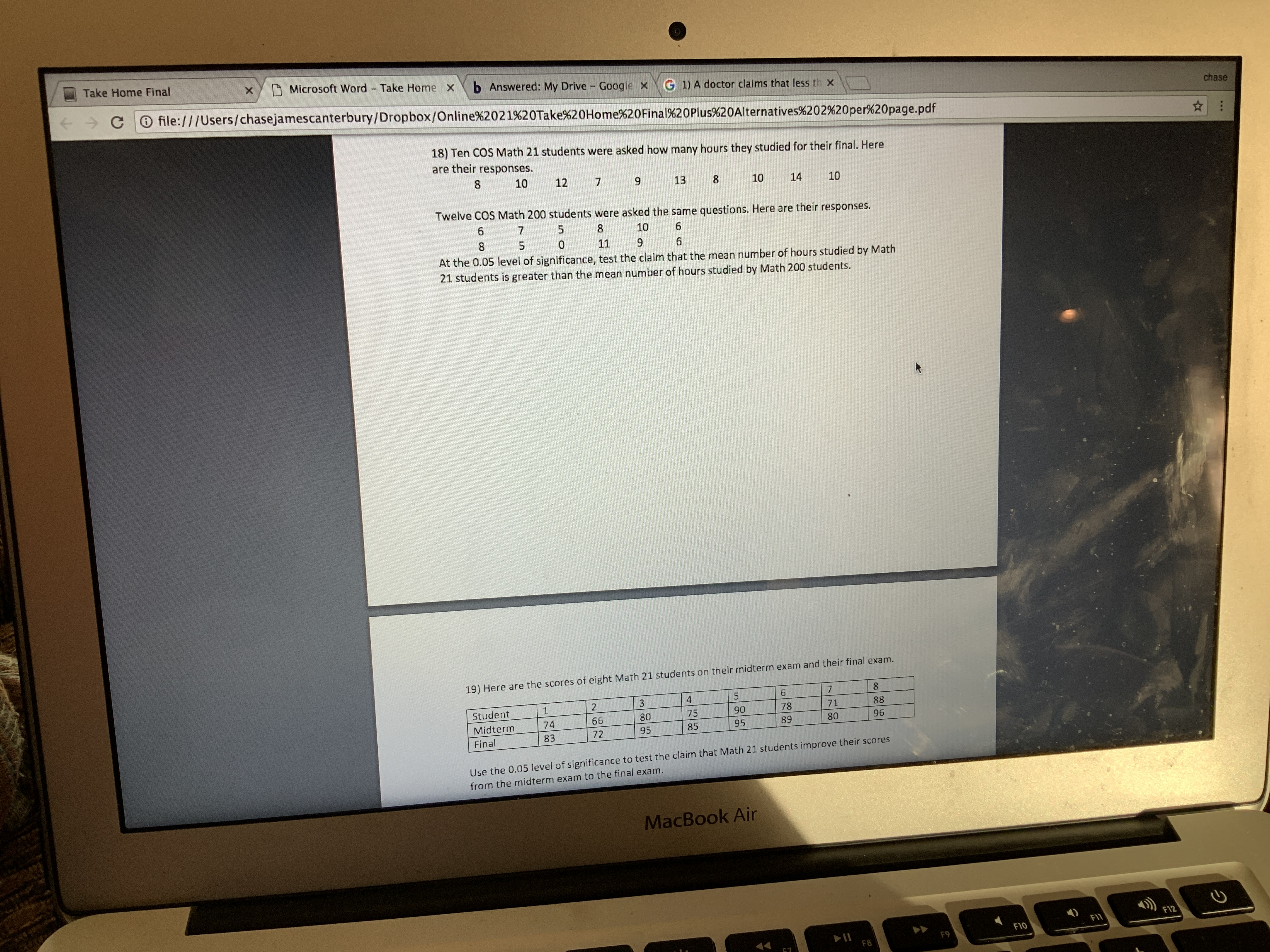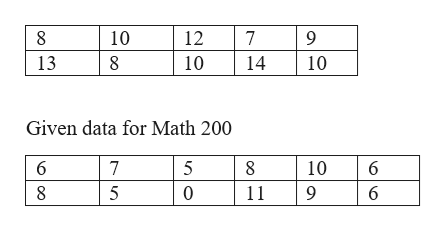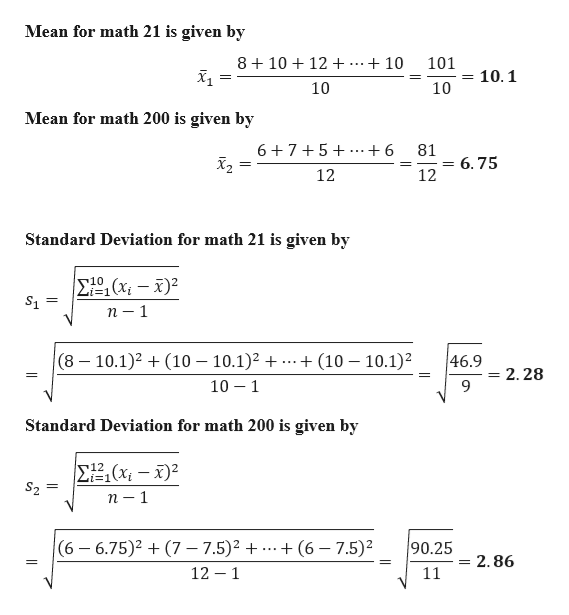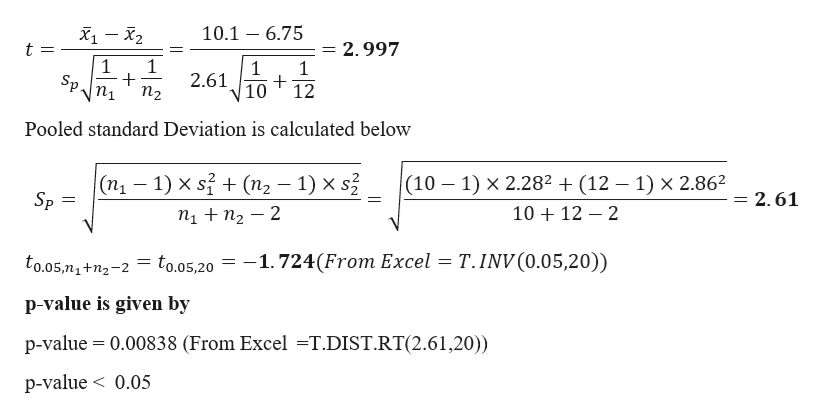# Take Home FinalMicrosoft Word Take Home xb Answered: My Drive Google XG 1) A doctor claims that less th xfile:///Users/chasejamescanterbury/Dropbox/Online%2021%20Take%20Home%20Final%20Plus%20Alternatives%202%20per%20page.pdfchase18) Ten COS Math 21 students were asked how many hours they studied for their final. Hereare their responses.101213101410Twelve COS Math 200 students were asked the same questions. Here are their responses.10119.At the 0.05 level of significance, test the claim that the mean number of hours studied by Math21 students is greater than the mean number of hours studied by Math 200 students.19) Here are the scores of eight Math 21 students on their midterm exam and their final exam.3Student8871789075806674Midterm8089958595729683FinalUse the 0.05 level of significance to test the claim that Math 21 students improve their scoresfrom the midterm exam to the final exam.MacBook AirF12F11>>F10F9F88.

Question
1 viewshelp_outlineImage TranscriptioncloseTake Home Final Microsoft Word Take Home x b Answered: My Drive Google X G 1) A doctor claims that less th x file:///Users/chasejamescanterbury/Dropbox/Online%2021%20Take%20Home%20Final%20Plus%20Alternatives%202%20per%20page.pdf chase 18) Ten COS Math 21 students were asked how many hours they studied for their final. Here are their responses. 10 12 13 10 14 10 Twelve COS Math 200 students were asked the same questions. Here are their responses. 10 11 9. At the 0.05 level of significance, test the claim that the mean number of hours studied by Math 21 students is greater than the mean number of hours studied by Math 200 students. 19) Here are the scores of eight Math 21 students on their midterm exam and their final exam. 3 Student 88 71 78 90 75 80 66 74 Midterm 80 89 95 85 95 72 96 83 Final Use the 0.05 level of significance to test the claim that Math 21 students improve their scores from the midterm exam to the final exam. MacBook Air F12 F11 >> F10 F9 F8 8. fullscreen
check_circle

Step 1

Given data for Math 21help_outlineImage Transcriptionclose10 12 13 10 14 10 Given data for Math 200 5 10 11 fullscreen
Step 2

Mean for math 21 is given byhelp_outlineImage TranscriptioncloseMean for math 21 is given by 8 + 10 + 12 + ..+ 10 101 10.1 10 10 Mean for math 200 is given by 6+7+5+...+6 81 = 6.75 12 12 Standard Deviation for math 21 is given by E(x; – x)² S1 Li%3D1 п — 1 + (10 – 10.1)2 46.9 (8 – 10.1)2 + (10 – 10.1)2 + ... 2.28 10 – 1 9. Standard Deviation for math 200 is given by E2,(x; – x)² S2 Li=1 п — 1 (6 – 6.75)2 + (7 – 7.5)2 + 12 – 1 + (6 – 7.5)² 90.25 ... = 2.86 11 fullscreen
Step 3

Formulation of Hypothesis

Null Hypothesis : There is no difference in the mean number of hours studied by math 21 students and math 200 students

H0: µ1 = µ2

Alternative Hypothesis: mean number of hours studied by math 21 students is greater th...help_outlineImage TranscriptioncloseX1 – X2 10.1 – 6.75 = 2.997 t = | 1 Sp. 2.61o+ 10 12 пi n2 Pooled standard Deviation is calculated below (10 – 1) x 2.28² + (12 – 1) × 2.86? (п, — 1) x s7 + (п, — 1) x s3 = 2.61 Sp 10 + 12 – 2 п + nz — 2 = to.05,20 = -1.724(From Excel = T. INV(0.05,20)) to.05,n1+n2-2 p-value is given by p-value = 0.00838 (From Excel =T.DIST.RT(2.61,20)) p-value < 0.05 fullscreen

### Want to see the full answer?

See Solution

#### Want to see this answer and more?

Solutions are written by subject experts who are available 24/7. Questions are typically answered within 1 hour.*

See Solution
*Response times may vary by subject and question.
Tagged in